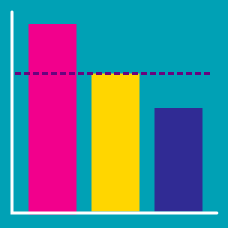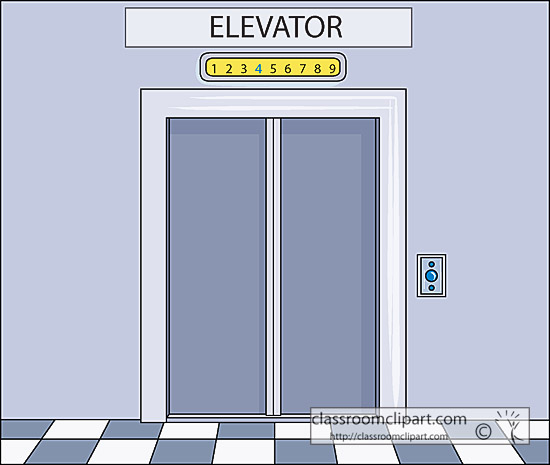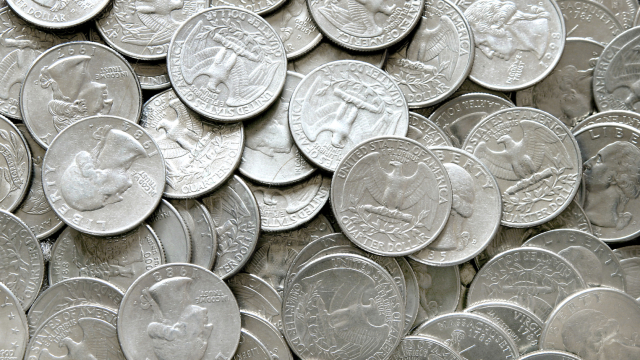Probability

# Expected Value: Level 5 ChallengesA particular elevator picks people up one at a time and brings them to their destination in the following manner:

1. The elevator starts at Floor 1
2. It travels to the floor of the person it needs to pick up
3. It brings the person to their desired floor
4. It returns to Floor 1

Consider the sequence of floors that an elevator stops on. If the building has 9 floors, and every person using the elevator has an equal chance of being on any floor and wanting to go to any other floor, then the average floor number that the elevator visits is $\frac{p}{q}$. If $p$ and $q$ are coprime integers, what is $p+q$?

Examples:

• Person is on Floor 4 and wants to go to Floor 7. The elevator starts at Floor 1 and goes to Floor 4, Floor 7, and then back to Floor 1.
• Person is on Floor 5 and wants to go to Floor 1. The elevator starts at Floor 1, goes to Floor 5, then goes back to Floor 1.

Notes:

• Assume that there is only one person waiting for the elevator at any given time.

• Assume that the number of people lining up to use this elevator is infinite.

Bonus: Can you find the general formula for N floors?

A class of 30 students contains 15 boys and 15 girls. Their classroom has 15 desks, and exactly 2 students can sit at each desk.

At the start of lessons one morning, the 30 students go into their classroom and seat themselves randomly, so that all possible arrangements of pupils are equally likely. The expected number of girls who end up sitting at a desk with another girl can be written as $\dfrac{a}{b}$, where $a$ and $b$ are coprime positive integers. What is the value of $a+b$?

Alice and Bob are playing a coin flipping game. Each of them will flip their own quarter 100 times and note the outcome of each flip on a line, in their own scoresheet: $H$ for heads and $T$ for tails.

If on any line Alice marks $HH$, she will give herself 1 point, begin a new line, and continue flipping.

If on any line Bob marks $HT$, he will give himself 1 point, begin a new line, and continue flipping.

After 100 flips each, who is more likely to have more points?

Clarification: Here is an example of a possible scoresheet for Alice after 25 flips, where she scores 3 points.

• $HTT \boxed{HH}$
• $HTTTTHTHTHTT \boxed{HH}$
• $TTT\boxed{HH}$
• $T$Taken from a numberphile video

I toss a fair coin 100 times in a row. If the expected number of times that two Heads are tossed consecutively is equal to $N$, what is the value of $100N$?

Clarification: A run of three or more Heads in a row will contribute more than one instance of consecutive Heads. If three Heads are tossed in a row, then two Heads have been tossed consecutively twice. If six Heads are tossed in a row, then two Heads have been tossed consecutively five times, and so on. For example, two Heads have been tossed consecutively 11 times in the following sequence of 30 tosses:

$THHTHHHHTHHHTHTHHHHHTTTTHHTHTT$

A row of street parking has spaces numbered 1 through 100, in that order. Each person trying to park drives by the spaces one-by-one starting at number 1.

At each open space, they are told how many open spaces, $S,$ they haven't passed (including the current space). They park in that space with probability $\frac{1}{S};$ otherwise, they move on to the next open space.

What is the expected value for the parking spot of the $100^\text{th}$ person to arrive (assuming no one has left and only one car goes through the parking process at a time)?

×